Next: ALL-PASS FILTERS Up: One-sided functions Previous: POSITIVE REAL FUNCTIONS

# NARROW-BAND FILTERS

Filters are often used to modify the spectrum of given data. With input X(Z), filters B(Z), and output Y(Z) we have Y(Z) = B(Z)X(Z) and the Fourier conjugate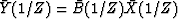. Multiplying these two relations together we get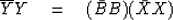(25)
which says that the spectrum of the input times the spectrum of the filter equals the spectrum of the output. Filters are often characterized by the shape of their spectra. Some examples are shown in Figure (13).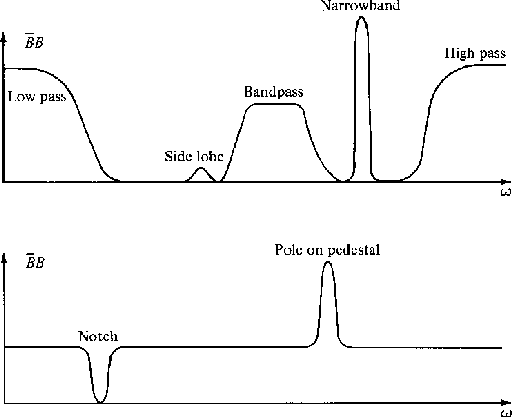2-13
Figure 13
Spectra of various filters.We will have frequent occasion to deal with sinusoidal time functions. A simple way to represent a sinusoid by Z transforms is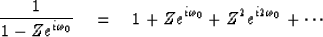(26)

The time function associated with this Z transform is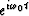, but it is turned on'' at t = 0. Actually, the left-hand side of (26) contains a pole exactly on the unit circle, so that the series sits on the borderline between convergence and divergence. This can cause paradoxical situations [you could expand (26) so that the sinusoid turns off at t = 0] which we will avoid by pushing the pole from the unit circle to a small distanceoutside the unit circle. Let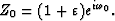Then define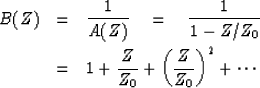(27)

The time function corresponding to B(Z) is zero before t = 0 and is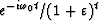after t = 0. It is a sinusoidal function which decreases gradually with time according to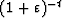.The coefficients are shown in Figure 14.

 2-14 Figure 14 The time function associated with a simple pole just outside the unit circle at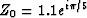.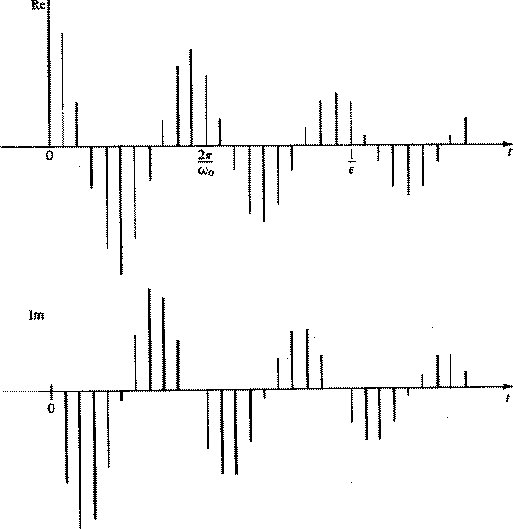It is intuitively obvious, although we will prove it later, that convolution with the coefficients of (27), which are sketched in Figure 14, is a narrow-banded filtering operation. If the pole is chosen very close to the unit circle, the filter bandpass becomes narrower and the coefficients of B(Z) drop off more and more slowly. To actually perform the convolution it is necessary to truncate, that is, to drop powers of Z beyond a certain practical limit. It turns out that there is a very much cheaper method of narrow-band filtering than convolution with the coefficients of B(Z). This method is polynomial division by A(Z). We have for the output Y(Z)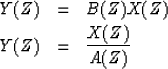(28) (29)
Multiply both sides of (29) by A(Z)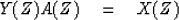(30)

For definiteness, let us suppose the xt and yt vanish before t = 0. Now identify coefficients of successive powers of Z. We get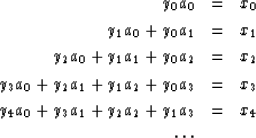(31)
A general equation is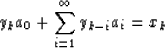(32)
Solving for yk we get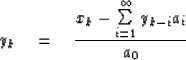(33)
Equation (33) may be used to solve for yk once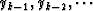are known. Thus the solution is recursive, and it will not diverge if the ai are coefficients of a minimum-phase polynomial. In practice the infinite limit on the sum is truncated whenever you run out of coefficients of either A(Z) or Y(Z). For the example we have been considering, B(Z) = 1/A(Z) = 1/(1 - Z/Z0), there will be only one term in the sum. Filtering in this way is called feedback filtering, and for narrowband filtering it will be vastly more economical than filtering by convolution, since there are much fewer coefficients in A(Z) than B(Z) = 1/A(Z). Finally, let us examine the spectrum of B(Z). We have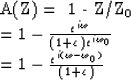and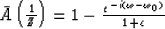so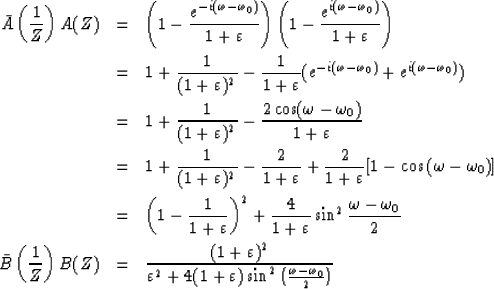(34)

To a good approximation this function may be thought of as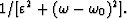A plot of (34) is shown in Figure 15.

 2-15 Figure 15 Spectrum associated with a single pole at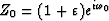.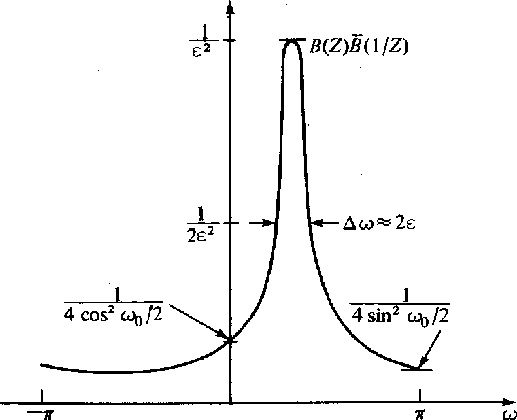Now it should be apparant why this is called a narrowband filter. It amplifies a vary narrow band of frequencies and attenuates all others. The frequency window of this filter is said to be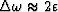in width. The time window is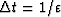,the damping time constant of the dampend sinusoid bt.

One practical disadvantage of the filter under discussion is that although its input may be a real time series its output will be a complex time series. For many applications a filter with real coefficients may be preferred.

One approach is to follow the filter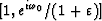by the time-domain, complex conjugate filter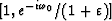.The composit time-domain operator is now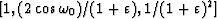which is real. [Note that the complex conjugate in the frequency domain is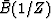but in the time domain it is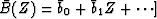.The composite filter may be denoted by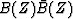.The spectrum of this filter is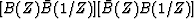.One may quickly verify that the spectrum of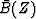is like that of B(Z), but the peak is at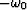instead of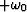.Thus, the composite spectrum is the product of Figure 15 with itself reversed along the frequency axis. This is shown in Figure 16.

 2-16 Figure 16 Spectrum of a two-pole filter where one pole is like Figure 15 and the other is at the conjugate position.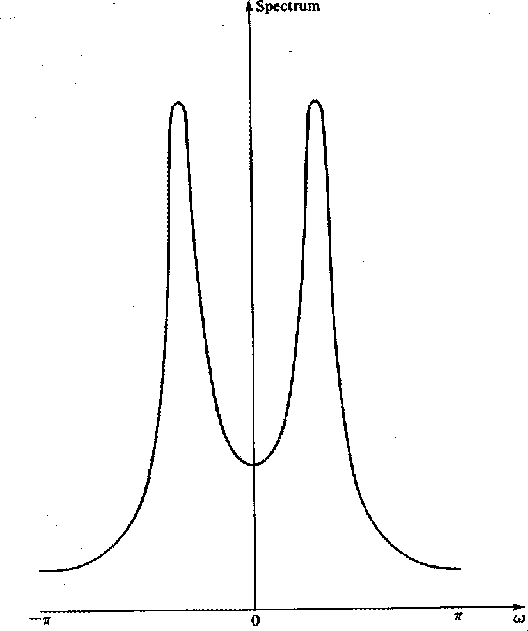## EXERCISES:

1. A simple feedback operation is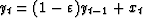.This operation is called leaky integration. Give a closed form expression for the output yt if xt is an impulse. What is the decay time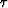of your solution (the time it takes for yt to drop to e-1y0)? For small,say = 0.1, .001, or 0.0001, what is?
2. How far from the unit circle are the poles of 1/(1 - .1Z + .9Z2)? What is the decay time of the filter and its resonant frequency?
3. Find a three-term real feedback filter to pass 59-61 Hz on data which are sampled at 500 points/sec. Where are the poles? What is the decay time of the filter?Next: ALL-PASS FILTERS Up: One-sided functions Previous: POSITIVE REAL FUNCTIONS
Stanford Exploration Project
10/30/1997